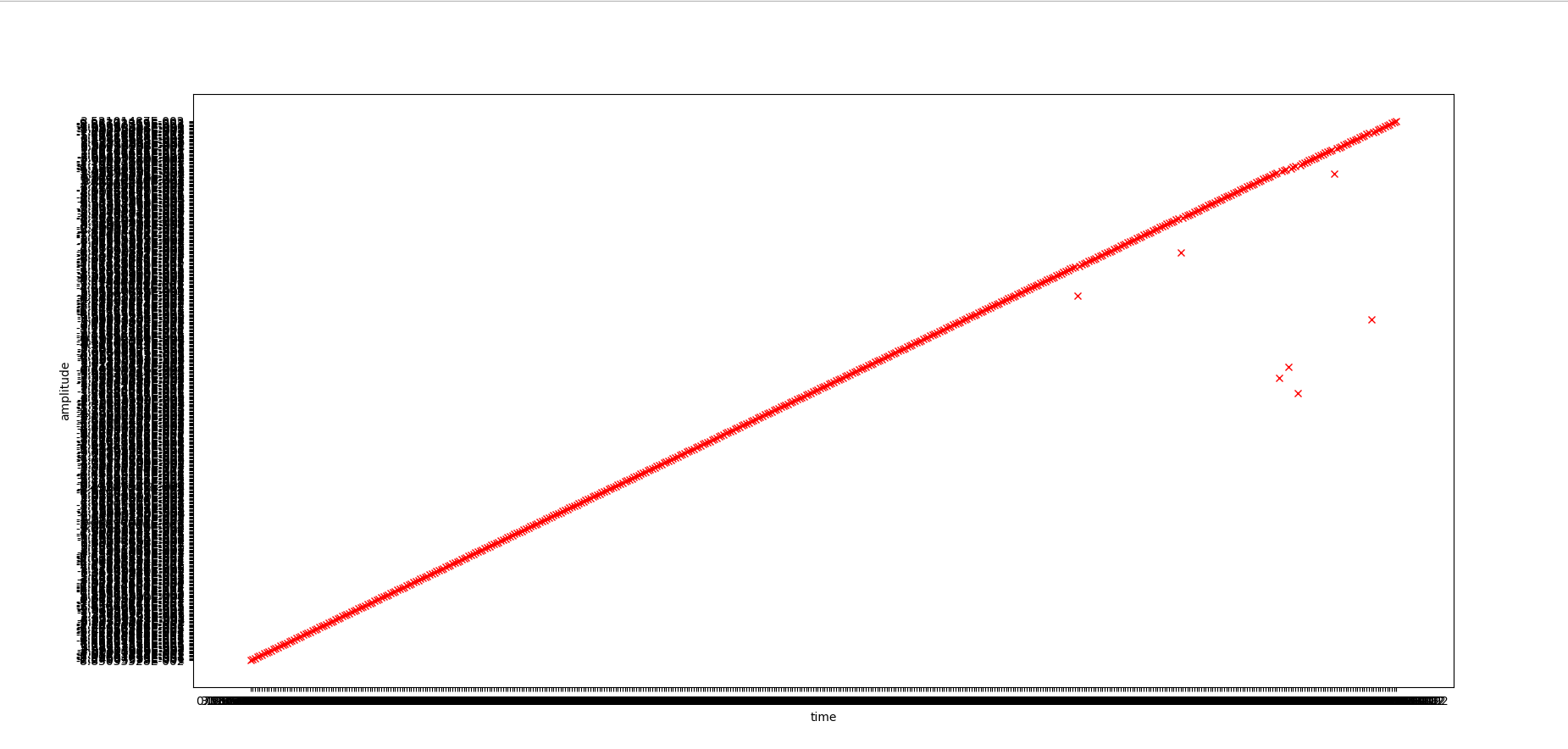###### tensor_zhang

2018-10-29 05:47 阅读 4.0k

# 如何用python读取txt文件中的科学计数表示的数据并绘图？

import matplotlib.pylab as pylab

def loaddata(filename):
infile = open(filename, 'r')

``````#定义两个list，用于存放数据
x = []
y = []

for line in infile:
trainingset = line.split()#对于每一行按照空格分开，将数据分为两部分。若用‘，’分隔，则写为line.split(',')
x.append(trainingset)#第一部分，即文件中的第一列数据逐一添加到list x中
y.append(trainingset)#第二部分，即文件中的第二列数据逐一添加到list y中

return(x, y) #x,y组成一个元组，通过函数一次性返回
``````

def plotdata(x, y):
length = len(y)
pylab.figure(1)

``````pylab.plot(x, y, 'rx')

pylab.xlabel('time')
pylab.ylabel('amplitude')

pylab.show()#让绘制的图形在屏幕上显示
``````

(x, y) = loaddata('test.txt')

plotdata(x, y)• 点赞
• 写回答
• 关注问题
• 收藏
• 复制链接分享

#### 1条回答默认 最新

• 研中经常需要作图和科学运算。如果有一个txt的数据文件，该如何导入Python中呢？比方说下面这种类型的数据文件：
wavelength,intenisty
400,325.2
401,356.9
402,369.8
...
这是一种以“，”分割的两列数据，另外还会经常遇到以Tab分割的数据，“ ”也就是“\t”；还可能会遇到多列数据的情况

点赞 1 评论 复制链接分享